﻿ BME - Yorick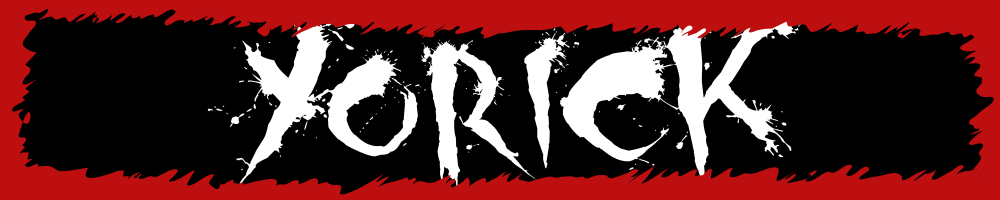# Biomedical Engineering

Below are some images and videos about BME stuff, mainly from my work in the cell and tissue engineering lab.

In a short experiment, migration of HeLa cells was investigated by means of a scratch assay. Using pipet tips, scratches were made on a monolayer of confluent HeLa cells. Migration of cells into the scratch was assessed after 6 and 14 hours. Different parts of the cytoskeleton were stained: F-actin was stained with TRITC-labeled phalloidin, vimentin (an intermediate filament protein) was stained using a specific antibody bound to Alexa 488. DAPI was used to stain nuclei. The images below were taken using a confocal microscope (Zeiss LSM510 META NLO).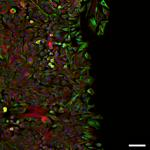t=6hM=10x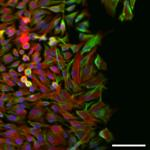t=6hM=20x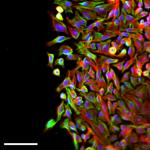t=6hM=20x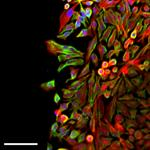t=6hM=20x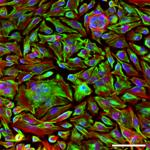t=6hM=20x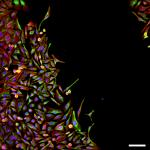t=14hM=10x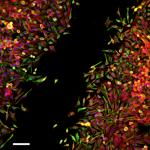t=14hM=10x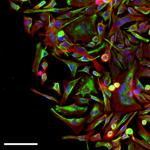t=14hM=20x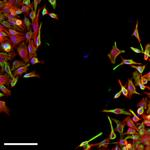t=14hM=20x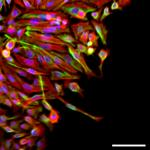t=14hM=20x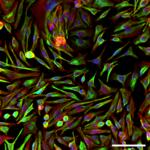t=14hM=20x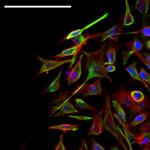t=14hM=40x

Next, some images of the same experiment taken with a normal fluorescence microscope (Zeiss Axiovert 200M).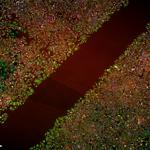t=6hM=4x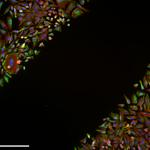t=6hM=10x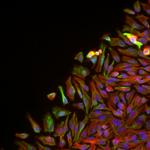t=6hM=20x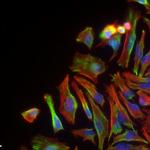t=6hM=40x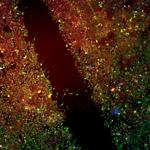t=14hM=4x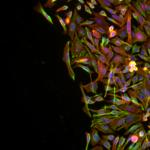t=14hM=20x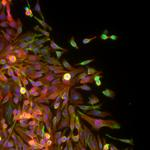t=14hM=20x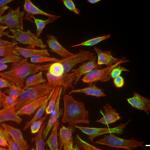t=14hM=40x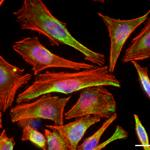t=14hM=63x

A video of human mesenchymal stem cells embedded in a collagen hydrogel. Live cells are stained green (calcein-AM), dead cell's nuclei are stained red (propidium iodide). Small green dots are fluorescent microbeads (ø1 um). Image slices were taken on a confocal microscope and converted into a video. Depth into the hydrogel (from bottom upwards) is displayed in the top left corner. Scale bar: 100 um.